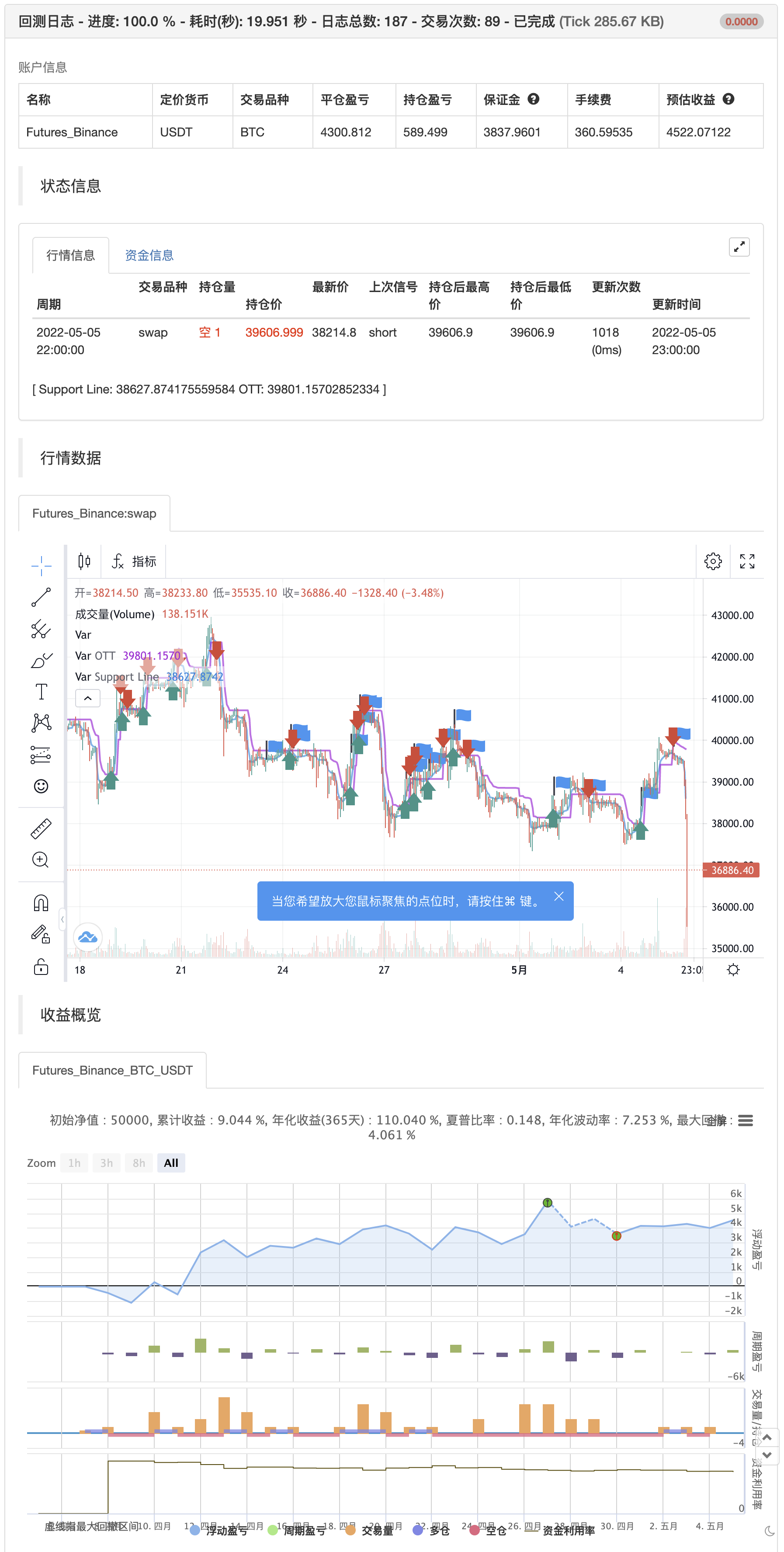# Optimized Trend Tracker

Author: 张超, Date: 2022-05-06 23:35:19
Tags: OTT

A brand new indicator from the developer of MOST (Moving Stop Loss) indicator Anıl Özekşi.

Optimized Trend Tracker OTT is an indicator that provides traders to find an existing trend or in another words to ser which side of the current trend we are on.

We are under the effect of the uptrend in cases where the prices are above OTT , under the influence of a downward trend, when prices are below OTT it is possible to say that we are.

The first parameter in the OTT indicator set by the two parameters is the period/length.

OTT will be much sensitive to trend movements if it is smaller. And vice versa, will be less sensitive when it is longer.

As the period increases it will become less sensitive to little trends and price actions.

In this way, your choice of period, will be closely related to which of the sort of trends you are interested in.

The OTT percent parameter in OTT is an optimization coefficient. Just like in the period small values ​​are better at capturing short term fluctuations, while large values will be more suitable for long-term trends.

In addition, when OTT is used with the support line in it, buy and sell signals it will become a producing indicator.

You can use OTT default alarms and Buy Sell signals like:

1- BUY when Prices are above OTT SELL when Prices are below OTT

2- BUY when OTT support Line crosses over OTT line. SELL when OTT support Line crosses under OTT line.

3- BUY when OTT line is Green and makes higher highs. SELL when OTT line is Red and makes lower lows.

Note: A small coverage with English subtitles will be available on my Youtube Channel soon. 回测```/*backtest
start: 2022-04-06 00:00:00
end: 2022-05-05 23:59:00
period: 1h
basePeriod: 15m
exchanges: [{"eid":"Futures_Binance","currency":"BTC_USDT"}]
*/
//@version=4
// This source code is subject to the terms of the Mozilla Public License 2.0 at https://mozilla.org/MPL/2.0/

//created by: @Anil_Ozeksi
//developer: ANIL ÖZEKŞİ
//author: @kivancozbilgic

study("Optimized Trend Tracker","OTT", overlay=true)
src = input(close, title="Source")
length=input(2, "OTT Period", minval=1)
percent=input(1.4, "OTT Percent", type=input.float, step=0.1, minval=0)
showsupport = input(title="Show Support Line?", type=input.bool, defval=true)
showsignalsk = input(title="Show Support Line Crossing Signals?", type=input.bool, defval=true)
showsignalsc = input(title="Show Price/OTT Crossing Signals?", type=input.bool, defval=false)
highlight = input(title="Show OTT Color Changes?", type=input.bool, defval=false)
showsignalsr = input(title="Show OTT Color Change Signals?", type=input.bool, defval=false)
highlighting = input(title="Highlighter On/Off ?", type=input.bool, defval=true)
mav = input(title="Moving Average Type", defval="VAR", options=["SMA", "EMA", "WMA", "TMA", "VAR", "WWMA", "ZLEMA", "TSF"])
Var_Func(src,length)=>
valpha=2/(length+1)
vud1=src>src ? src-src : 0
vdd1=src<src ? src-src : 0
vUD=sum(vud1,9)
vDD=sum(vdd1,9)
vCMO=nz((vUD-vDD)/(vUD+vDD))
VAR=0.0
VAR:=nz(valpha*abs(vCMO)*src)+(1-valpha*abs(vCMO))*nz(VAR)
VAR=Var_Func(src,length)
Wwma_Func(src,length)=>
wwalpha = 1/ length
WWMA = 0.0
WWMA := wwalpha*src + (1-wwalpha)*nz(WWMA)
WWMA=Wwma_Func(src,length)
Zlema_Func(src,length)=>
zxLag = length/2==round(length/2) ? length/2 : (length - 1) / 2
zxEMAData = (src + (src - src[zxLag]))
ZLEMA=Zlema_Func(src,length)
Tsf_Func(src,length)=>
lrc = linreg(src, length, 0)
lrc1 = linreg(src,length,1)
lrs = (lrc-lrc1)
TSF = linreg(src, length, 0)+lrs
TSF=Tsf_Func(src,length)
getMA(src, length) =>
ma = 0.0
if mav == "SMA"
ma := sma(src, length)
ma

if mav == "EMA"
ma := ema(src, length)
ma

if mav == "WMA"
ma := wma(src, length)
ma

if mav == "TMA"
ma := sma(sma(src, ceil(length / 2)), floor(length / 2) + 1)
ma

if mav == "VAR"
ma := VAR
ma

if mav == "WWMA"
ma := WWMA
ma

if mav == "ZLEMA"
ma := ZLEMA
ma

if mav == "TSF"
ma := TSF
ma
ma

MAvg=getMA(src, length)
fark=MAvg*percent*0.01
longStop = MAvg - fark
longStopPrev = nz(longStop, longStop)
longStop := MAvg > longStopPrev ? max(longStop, longStopPrev) : longStop
shortStop =  MAvg + fark
shortStopPrev = nz(shortStop, shortStop)
shortStop := MAvg < shortStopPrev ? min(shortStop, shortStopPrev) : shortStop
dir = 1
dir := nz(dir, dir)
dir := dir == -1 and MAvg > shortStopPrev ? 1 : dir == 1 and MAvg < longStopPrev ? -1 : dir
MT = dir==1 ? longStop: shortStop
OTT=MAvg>MT ? MT*(200+percent)/200 : MT*(200-percent)/200
plot(showsupport ? MAvg : na, color=#0585E1, linewidth=2, title="Support Line")
OTTC = highlight ? OTT > OTT ? color.green : color.red : #B800D9
pALL=plot(nz(OTT), color=OTTC, linewidth=2, title="OTT", transp=0)

sellSignallk = crossunder(MAvg, OTT)
plotshape(sellSignallk and showsignalsk ? OTT*1.005 : na, title="Sell", text="Sell", location=location.absolute, style=shape.labeldown, size=size.tiny, color=color.red, textcolor=color.white, transp=0)